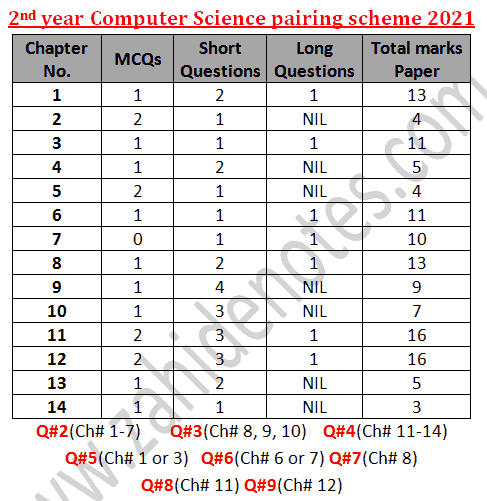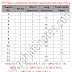Now for various reasons, I got late to post the paper schemes of computer science for 2nd year 2021, ics part 2 students. You can find the scheme here on this page as you will be able to get it in pdf too. Currently I have posted it here as an image and as texts.

# 2nd year computer science pairing scheme 2021

I could not remember whether the students like to study according to the paper scheme or they just cram the whole book or they want to clear their concepts of computer science for 2nd year. The paper scheme gives you an idea of how the paper will be made keeping in view the importance of an weightage of different chapters.

## 2nd year class 12 computer science Paper scheme 2021

The paper scheme and pairing scheme of computer 2021 is given below for all punjab boards.If you cannot download the image or pdf version of 2021 paper scheme, you can take screenshots. You an also copy the texts to your phone. The paper scheme for ICS part 2 computer science 2021 is similar to that of 2020. But there are some 2 or 3 minor changes in the new pairing scheme.

Question No. 1 MCQs = 15(1 from chapter 1, 3, 4, 6, 8, 9, 10, 13, 14 each and 2 mcqs from chapter 2, 5, 11, 12 each)

Short questions

Question No. 2 (6/9)

Chapter 1 = 2
Chapter 2 = 1
Chapter 3 = 1
Chapter 4 = 2
Chapter 5 = 1
Chapter 6 = 1
Chapter 7 = 1

Question No. 3 (6/9)
Chapter 8 = 2
Chapter 9 = 4
Chapter 10 = 3

Question No. 4 (6/9)
Chapter 11 = 3 (Find error/output of code)
Chapter 12 = 3 ( 1 output, 1 conversion)
Chapter 13 = 2
Chapter 14 = 1

Long questions (Attempt ANY THREE questions from FIVE Questions)

Questions No. 5
Chapter 1 OR 3

Questions No. 6

Chapter 6 OR 7

Questions No. 7
Chapter 8 (theory)

Questions No. 8
Chapter 11 (theory)

Questions No. 9
Chapter 12 (program)

Zahid notes has currently published new short questions notes for 2nd year ICS part 2 computer science. You can download computer science notes for 2nd year class 12. To download the notes visit 2nd year computer notes page and click download button.

2nd year computer scheme 2021Reviewed by Saif Ullah ZahidonJanuary 26, 2021 Rating: 5

1.Total marks 113 bnty hain???? Kese

1.bhai choice hoti hai

2.BHAI TOTAL MARKS 85 H. YEH CHOICE WALY QUESTION DL K MARKS BANTY H

3.Kuchh MCQs ki choice hoti hai

2.kya MCQS baise py aye ga paper?

3.ye short syllabus k lehaz se bni hui ha?

4.Ye fake to ney ha sir